# Selina solutions for Concise Mathematics Class 8 ICSE chapter 6 - Sets [Latest edition]

#### Chapters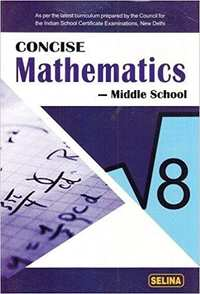## Solutions for Chapter 6: Sets

Below listed, you can find solutions for Chapter 6 of CISCE Selina for Concise Mathematics Class 8 ICSE.

Exercise 6 (A)Exercise 6 (B)Exercise 6 (C)Exercise 6 (D)Exercise 6 (E)
Exercise 6 (A) [Page 65]

### Selina solutions for Concise Mathematics Class 8 ICSE Chapter 6 Sets Exercise 6 (A) [Page 65]

Exercise 6 (A) | Q 1.1 | Page 65

Write the following sets in roster (Tabular) form :
A1 = {x : 2x + 3 = 11}

Exercise 6 (A) | Q 1.2 | Page 65

Write the following sets in roster (Tabular) form :
A2 = {x : x2 - 4x -5 = 0}

Exercise 6 (A) | Q 1.3 | Page 65

Write the following sets in roster (Tabular) form:

A3 = {x : x ∈ Z, -3 ≤ x <4}

Exercise 6 (A) | Q 1.4 | Page 65

Write the following sets in roster (Tabular) form :

A4 = {x : x is a two digit number and sum of digits of x is 7}

Exercise 6 (A) | Q 1.5 | Page 65

Write the following sets in roster (Tabular) form :

A5 = {x : x = 4n, n ∈ W and n < 4}

Exercise 6 (A) | Q 1.6 | Page 65

Write the following sets in roster (Tabular) form :
A6 = {x : x = n/(n+2); n ∈ N and n > 5}

Exercise 6 (A) | Q 2.1 | Page 65

Write the following sets in set-builder (Rule Method) form:

B1 = {6, 9, 12, 15 ....}

Exercise 6 (A) | Q 2.2 | Page 65

Write the following sets in set-builder (Rule Method) form :

B2 = {11, 13, 7,  19}

Exercise 6 (A) | Q 2.3 | Page 65

Write the following sets in set-builder (Rule Method) form :

B3 = {1/3, 3/5, 5/7, 7/9, 9/11, ....}

Exercise 6 (A) | Q 2.4 | Page 65

Write the following sets in set-builder (Rule Method) form :
B4 = {8, 27, 64, 125, 216}

Exercise 6 (A) | Q 2.5 | Page 65

Write the following sets in set-builder (Rule Method) form :
B5 = {-5, -4, -3, -2, -1}

Exercise 6 (A) | Q 2.6 | Page 65

Write the following sets in set-builder (Rule Method) form :
B6 = {....., -6, -3, 0, 3, 6 ......}

Exercise 6 (A) | Q 3.1 | Page 65

Is {1, 2, 4, 16, 64} = {x : x is a factor of 32}? Give reason.

Exercise 6 (A) | Q 3.2 | Page 65

Is {x : x is a factor of 27} ≠ {3, 9, 27, 54} ? Give reason.

Exercise 6 (A) | Q 3.3 | Page 65

Write the set of even factors of 124.

Exercise 6 (A) | Q 3.4 | Page 65

Write the set of odd factors of 72.

Exercise 6 (A) | Q 3.5 | Page 65

Write the set of prime factors of 3234.

Exercise 6 (A) | Q 3.6 | Page 65

Is {x : x2 – 7x + 12 = 0} = {3, 4} ?

Exercise 6 (A) | Q 3.7 | Page 65

Is {x : x2 – 5x – 6 = 0} = {2, 3} ?

Exercise 6 (A) | Q 4.1 | Page 65

Write the following sets in Roster form:

The set of letters in the word ‘MEERUT’

Exercise 6 (A) | Q 4.2 | Page 65

Write the following sets in Roster form:

The set of letters in the word ‘UNIVERSAL’.

Exercise 6 (A) | Q 4.3 | Page 65

Write the following sets in Roster form:

A = {x : x = y + 3, y ∈N and y > 3}

Exercise 6 (A) | Q 4.4 | Page 65

Write the following sets in Roster form:

B = {p : p ∈ W and p2 < 20}

Exercise 6 (A) | Q 4.5 | Page 65

Write the following sets in Roster form:

C = {x : x is composite number and 5 ≤ x ≤ 21}

Exercise 6 (A) | Q 5.1 | Page 65

List the elements of the following sets:

{x : x2 – 2x – 3 = 0}

Exercise 6 (A) | Q 5.2 | Page 65

List the elements of the following sets:

{x : x = 2y + 5; y ∈ N and 2 ≤ y < 6}

Exercise 6 (A) | Q 5.3 | Page 65

List the elements of the following sets:

{x : x is a factor of 24}

Exercise 6 (A) | Q 5.4 | Page 65

List the elements of the following sets:

{x : x ∈ Z and x2 ≤ 4}

Exercise 6 (A) | Q 5.5 | Page 65

List the elements of the following sets:

{x : 3x – 2 ≤ 10, x ∈ N}

Exercise 6 (A) | Q 5.6 | Page 65

List the elements of the following sets:

{x : 4 – 2x > -6, x ∈ Z}

Exercise 6 (B) [Pages 67 - 68]

### Selina solutions for Concise Mathematics Class 8 ICSE Chapter 6 Sets Exercise 6 (B) [Pages 67 - 68]

Exercise 6 (B) | Q 1.1 | Page 67

Find the cardinal number of the following sets:

A1 = {-2, -1, 1, 3, 5}

Exercise 6 (B) | Q 1.2 | Page 67

Find the cardinal number of the following sets:

A2 = {x : x ∈ N and 3 ≤ x <7}

Exercise 6 (B) | Q 1.3 | Page 67

Find the cardinal number of the following sets:

A3 = {p : p ∈ W and 2P - 3 < 8}

Exercise 6 (B) | Q 1.4 | Page 67

Find the cardinal number of the following sets:

A4 = {b : b ∈ Z and -7 < 3b -1 ≤ 2}

Exercise 6 (B) | Q 2 | Page 67

If P = {P : P is a letter in the word “PERMANENT”}. Find n (P).

Exercise 6 (B) | Q 3.1 | Page 67

State the following sets are finite or infinite:

A = {x : x ∈ Z and x < 10}

Exercise 6 (B) | Q 3.2 | Page 67

State the following sets are finite or infinite:

B = {x : x ∈ W and 5x -3 ≤ 20}

Exercise 6 (B) | Q 3.3 | Page 67

State the following sets are finite or infinite:

P = {y : y = 3x -2, x ∈ N & x > 5}

Exercise 6 (B) | Q 3.4 | Page 67

State the following sets are finite or infinite:

M = {r : r = 3/"n"; n ∈ W and 6 < n ≤ 15}

Exercise 6 (B) | Q 4.1 | Page 67

Find, if the following sets are singleton sets:

The set of points of intersection of two non-parallel st. lines in the same plane

Exercise 6 (B) | Q 4.2 | Page 67

Find, if the following sets are singleton sets:

A = {x : 7x – 3 = 11}

Exercise 6 (B) | Q 4.3 | Page 67

Find, if the following sets are singleton sets:

B = {y : 2y + 1 < 3 and y ∈ W}

Exercise 6 (B) | Q 5.1 | Page 67

Find, if the following sets are empty:

The set of points of intersection of two parallel lines.

Exercise 6 (B) | Q 5.2 | Page 67

Find, if the following sets are empty:

A = {x : x ∈ N and 5 < x < 6}

Exercise 6 (B) | Q 5.3 | Page 67

Find, if the following sets are empty:

B = {x : x2 + 4 = 0, x ∈ N}

Exercise 6 (B) | Q 5.4 | Page 67

Find, if the following sets are empty:

C = {even numbers between 6 & 10}

Exercise 6 (B) | Q 5.5 | Page 67

Find, if the following sets are empty:

D = {prime numbers between 7 & 11}

Exercise 6 (B) | Q 6.1 | Page 67

Are the sets A = {4, 5, 6} and B = {x : x2 – 5x – 6 = 0} disjoint?

Exercise 6 (B) | Q 6.2 | Page 67

Are the sets A = {b, c, d, e} and B = {x : x is a letter in the word ‘MASTER’} joint?

Exercise 6 (B) | Q 7.1 | Page 67

State, if the following pair of a set is equivalent or not:

A = {x : x ∈ N and 11 ≥ 2x – 1} and B = {y : y ∈ W and 3 ≤ y ≤ 9}

Exercise 6 (B) | Q 7.2 | Page 67

State, if the following pair of a set is equivalent or not:

Set of integers and set of natural numbers.

Exercise 6 (B) | Q 7.3 | Page 67

State, if the following pair of a set is equivalent or not:

Set of whole numbers and set of multiples of 3.

Exercise 6 (B) | Q 7.4 | Page 67

State, if the following pair of a set is equivalent or not:

P = {5, 6, 7, 8} and M = {x : x ∈ W and x < 4}

Exercise 6 (B) | Q 8.1 | Page 67

State, if the following pair of a set is equal or not :

A = {2, 4, 6, 8} and B = {2n : n ∈ N and n < 5}

Exercise 6 (B) | Q 8.2 | Page 67

State, if the following pair of a set is equal or not :

M = {x : x ∈ W and x + 3 < 8} and N = {y : y = 2n -1, n ∈ N and n < 5}

Exercise 6 (B) | Q 8.3 | Page 67

State, if the following pair of a set is equal or not:

E = {x : x 2 + 8x - 9 = 0} and F = {1, - 9}

Exercise 6 (B) | Q 8.4 | Page 67

State, if the following pair of a set is equal or not:

A = {x : x ∈ N, x < 3} and B = {y : y2 - 3y + 2 = 0}

Exercise 6 (B) | Q 9.1 | Page 67

State if the following set is a finite set or an infinite set:

The set of multiples of 8.

Exercise 6 (B) | Q 9.2 | Page 67

State if the following set is a finite set or an infinite set:

The set of integers less than 10.

Exercise 6 (B) | Q 9.3 | Page 67

State if the following set is a finite set or an infinite set:

The set of whole numbers less than 12.

Exercise 6 (B) | Q 9.4 | Page 67

State if the following set is a finite set or an infinite set:

{x : x = 3n – 2, n ∈ W, n ≤ 8}

Exercise 6 (B) | Q 9.5 | Page 67

State if the following set is a finite set or an infinite set:

{x : x = 3n – 2,n ∈ Z, n ≤ 8}

Exercise 6 (B) | Q 9.6 | Page 67

State if the following set is a finite set or an infinite set:

{x : x = (n-2)/(n+2), n ∈ w)

Exercise 6 (B) | Q 10.1 | Page 68

State the following statement is true or false:

The set of even natural numbers less than 21 and the set of odd natural numbers less than 21 are equivalent sets.

• True

• False

Exercise 6 (B) | Q 10.2 | Page 68

State the following statement is true or false:

If E = {factors of 16} and F = {factors of 20}, then E = F.

• True

• False

Exercise 6 (B) | Q 10.3 | Page 68

State the following statement is true or false:

The set A = {integers less than 20} is a finite set.

• True

• False

Exercise 6 (B) | Q 10.4 | Page 68

State the following statement is true or false:

If A = {x : x is an even prime number}, then set A is empty.

• True

• False

Exercise 6 (B) | Q 10.5 | Page 68

State the following statement is true or false:

The set of odd prime numbers is the empty set.

• True

• False

Exercise 6 (B) | Q 10.6 | Page 68

State the following statement is true or false:

The set of squares of integers and the set of whole numbers are equal sets.

• True

• False

Exercise 6 (B) | Q 10.7 | Page 68

State the following statement is true or false:

In n(P) = n(M), then P → M.

• True

• False

Exercise 6 (B) | Q 10.8 | Page 68

State the following statement is true or false:

If set P = set M, then n(P) = n(M).

• True

• False

Exercise 6 (B) | Q 10.9 | Page 68

State the following statement is true or false:

n(A) = n(B) => A = B.

• True

• False

Exercise 6 (C) [Pages 70 - 71]

### Selina solutions for Concise Mathematics Class 8 ICSE Chapter 6 Sets Exercise 6 (C) [Pages 70 - 71]

Exercise 6 (C) | Q 1.1 | Page 70

Find the subset of the following set:

A = {5, 7}

Exercise 6 (C) | Q 1.2 | Page 70

Find the subset of the following set:

B = {a, b, c}

Exercise 6 (C) | Q 1.3 | Page 70

Find the subset of the following set:

C = {x : x ∈ W, x ≤ 2}

Exercise 6 (C) | Q 1.4 | Page 70

Find the subset of the following set:

{p : p is a letter in the word ‘poor’}

Exercise 6 (C) | Q 2.1 | Page 70

If C is the set of letters in the word “cooler”, find: Set C

Exercise 6 (C) | Q 2.2 | Page 70

If C is the set of letters in the word “cooler”, find: n(C)

Exercise 6 (C) | Q 2.3 | Page 70

If C is the set of letters in the word “cooler”, find: The number of its subsets.

Exercise 6 (C) | Q 2.4 | Page 70

If C is the set of letters in the word “cooler”, find: Number of its proper subsets.

Exercise 6 (C) | Q 3 | Page 70

If T = {x : x is a letter in the word ‘TEETH’}, find all its subsets.

Exercise 6 (C) | Q 4.1 | Page 70

Given the universal set = {-7,-3, -1, 0, 5, 6, 8, 9}, find: A = {x : x < 2}

Exercise 6 (C) | Q 4.2 | Page 70

Given the universal set = {-7,-3, -1, 0, 5, 6, 8, 9}, find: B = {x : -4 < x < 6}

Exercise 6 (C) | Q 5.1 | Page 70

Given the universal set = {x : x ∈ N and x < 20}, find :
A = {x : x = 3p ; p ∈ N}

Exercise 6 (C) | Q 5.2 | Page 70

Given the universal set = {x : x ∈ N and x < 20}, find:

B = {y : y = 2n + 3, n ∈ N}

Exercise 6 (C) | Q 5.3 | Page 70

Given the universal set = {x : x ∈ N and x < 20}, find:

C = {x : x is divisible by 4}

Exercise 6 (C) | Q 6 | Page 70

Find the proper subsets of {x : x2 – 9x – 10 = 0}

Exercise 6 (C) | Q 7.1 | Page 70

Given, A = {Triangles}, B = {Isosceles triangles}, C = {Equilateral triangles}. State the following statement is true or false. Give reasons.
A ⊂ B

• True

• False

Exercise 6 (C) | Q 7.2 | Page 70

Given, A = {Triangles}, B = {Isosceles triangles}, C = {Equilateral triangles}. State the following statement is true or false. Give reasons.

B ⊆ A

• True

• False

Exercise 6 (C) | Q 7.3 | Page 70

Given, A = {Triangles}, B = {Isosceles triangles}, C = {Equilateral triangles}. State the following statement is true or false. Give reasons.

C ⊆ B

• True

• False

Exercise 6 (C) | Q 7.4 | Page 70

Given, A = {Triangles}, B = {Isosceles triangles}, C = {Equilateral triangles}. State the following statment is true or false. Give reasons.

B ⊂ A

• True

• False

Exercise 6 (C) | Q 7.5 | Page 70

Given, A = {Triangles}, B = {Isosceles triangles}, C = {Equilateral triangles}. State the following statement is true or false. Give reasons.

C ⊂ A

• True

• False

Exercise 6 (C) | Q 7.6 | Page 70

Given, A = {Triangles}, B = {Isosceles triangles}, C = {Equilateral triangles}. State the following statement is true or false. Give reasons.

C ⊆ B ⊆ A

• True

• False

Exercise 6 (C) | Q 8.1 | Page 70

Given, A = {Quadrilaterals}, B = {Rectangles}, C = {Squares}, D= {Rhombuses}. State the following statement is true or false. Give reasons.

B ⊂ C

• True

• False

Exercise 6 (C) | Q 8.2 | Page 70

Given, A = {Quadrilaterals}, B = {Rectangles}, C = {Squares}, D= {Rhombuses}. State the following statement is true or false. Give reasons.

D ⊂ B

• True

• False

Exercise 6 (C) | Q 8.3 | Page 70

Given, A = {Quadrilaterals}, B = {Rectangles}, C = {Squares}, D= {Rhombuses}. State the following statement is true or false. Give reasons.

C ⊆ B ⊆ A

• True

• False

Exercise 6 (C) | Q 8.4 | Page 70

Given, A = {Quadrilaterals}, B = {Rectangles}, C = {Squares}, D= {Rhombuses}. State the following statement is true or false. Give reasons.

D ⊂ A

• True

• False

Exercise 6 (C) | Q 8.5 | Page 70

Given, A = {Quadrilaterals}, B = {Rectangles}, C = {Squares}, D= {Rhombuses}. State the following statement is true or false. Give reasons.
B ⊇ C

• True

• False

Exercise 6 (C) | Q 8.6 | Page 70

Given, A = {Quadrilaterals}, B = {Rectangles}, C = {Squares}, D= {Rhombuses}. State the following statement is true or false. Give reasons.

A ⊇ B ⊇ D

• True

• False

Exercise 6 (C) | Q 9.1 | Page 70

Given, universal set = {x : x ∈ N, 10 ≤ x ≤  35}.
A = {x ∈ N : x ≤ 16} Find: A'

Exercise 6 (C) | Q 9.2 | Page 70

Given, universal set = {x : ∈ N, 10 ≤ x ≤ 35}.
B = {x : x > 29} Find: B'.

Exercise 6 (C) | Q 10.1 | Page 71

Given universal set = {x ∈ Z : -6 < x ≤6}.
N = {n : n is non-negative number}
Find: N'

Exercise 6 (C) | Q 10.2 | Page 71

Given universal set = {x ∈ Z : -6 < x ≤ 6}. P = {x : x is a non-positive number}. Find: P'

Exercise 6 (C) | Q 11.1 | Page 71

Let M = {letters of the word REAL} and N = {letters of the word LARE}. Write sets M and N in roster form and then state whether;
M ⊆ N is true.

Exercise 6 (C) | Q 11.2 | Page 71

Let M = {letters of the word REAL} and N = {letters of the word LARE}. Write sets M and N in roster form and then state whether
N ⊆ M is true.

Exercise 6 (C) | Q 11.3 | Page 71

Let M = {letters of the word REAL} and N = {letters of the word LARE}. Write sets M and N in roster form and then state whether
M = N is true.

Exercise 6 (C) | Q 12 | Page 71

Write two sets A and B such that A ⊆ B and B ⊆ A.State the relationship between sets A and B.

Exercise 6 (D) [Pages 72 - 73]

### Selina solutions for Concise Mathematics Class 8 ICSE Chapter 6 Sets Exercise 6 (D) [Pages 72 - 73]

Exercise 6 (D) | Q 1.1 | Page 72

Given A = {x : x ∈ N and 3 < x ≤ 6} and B = {x : x ∈ W and x < 4}. Find : Sets A and B in roster form.

Exercise 6 (D) | Q 1.2 | Page 72

Given A = {x : x ∈ N and 3 < x ~ 6} and 8 = {x : x ∈ W and x < 4}. Find: A ∪ B

Exercise 6 (D) | Q 1.3 | Page 72

Given A = {x : x ∈ N and 3 < x ~ 6} and 8 = {x : x ∈ W and x < 4}. Find: A ∩ B.

Exercise 6 (D) | Q 1.4 | Page 72

Given A = {x : x ∈ N and 3 < x ~ 6} and 8 = {x : x ∈ W and x < 4}. Find: A - B.

Exercise 6 (D) | Q 1.5 | Page 72

Given A = {x : x ∈ N and 3 < x ~ 6} and 8 = {x : x ∈ W and x < 4}. Find: B - A.

Exercise 6 (D) | Q 2.1 | Page 72

If P = {x : x ∈ W and 4 ≤ x ≤ 8}, and Q = {x : x ∈ N and x < 6}. Find: P ∪ Q and P ∩  Q.

Exercise 6 (D) | Q 2.2 | Page 72

If P = {x : x ∈ W and 4 ≤ x ≤ 8}, and Q = {x : x ∈ N and x < 6}. Find: Is (P ∪ Q) ⊃ (P ∩ Q)?

Exercise 6 (D) | Q 3.1 | Page 72

If A = {5, 6, 7, 8, 9}, B = {x : 3 < x < 8 and x ∈ W} and C = {x : x ≤ 5 and x ∈ N}.
Find: A ∪ B and (A ∪ B) ∪ C

Exercise 6 (D) | Q 3.2 | Page 72

If A = {5, 6, 7, 8, 9}, B = {x : 3 < x < 8 and x ∈ W} and C = {x : x ≤ 5 and x ∈ N}. Find:

B ∪ C and A ∪ (B ∪ C)

Exercise 6 (D) | Q 3.3 | Page 72

If A = {5, 6, 7, 8, 9}, B = {x : 3 < x < 8 and x ∈ W} and C = {x : x ≤ 5 and x ∈ N}. Find:
A ∩ B and (A ∩ B) ∩ C

Exercise 6 (D) | Q 3.4 | Page 72

If A = {5, 6, 7, 8, 9}, B = {x : 3 < x < 8 and x ∈ W} and C = {x : x ≤ 5 and x ∈ N}. Find:

B ∩ C and A ∩ (B ∩  C)
Is (A ∪ B) ∪ C = A ∪ (B ∪ C)?
Is (A ∩ B) ∩ C = A ∩ (B ∩ C)?

Exercise 6 (D) | Q 4.1 | Page 72

Given A = {0, 1, 2, 4, 5}, B = {0, 2, 4, 6, 8} and C = {0, 3, 6, 9}. Show that  A ∪ (B ∪ C) = (A ∪ B) ∪ C i.e. the union of sets is associative.

Exercise 6 (D) | Q 4.2 | Page 72

Given A = {0, 1, 2, 4, 5}, B = {0, 2, 4, 6, 8} and C = {0, 3, 6, 9}. Show that  A ∩ (B ∩ C) = (A ∩ B) ∩ C i.e. the intersection of sets is associative.

Exercise 6 (D) | Q 5.1 | Page 73

If A = {x ∈ W : 5 < x < 10}, B = {3, 4, 5, 6, 7} and C = {x = 2n; n ∈ N and n ≤4}. Find:

A ∩ (B ∪ C)

Exercise 6 (D) | Q 5.2 | Page 73

If A = {x ∈ W : 5 < x < 10}, B = {3, 4, 5, 6, 7} and C = {x = 2n; n ∈ N and n ≤4}. Find:

(B ∪ A) ∩  (B ∪ C)

Exercise 6 (D) | Q 5.3 | Page 73

If A = {x ∈ W : 5 < x < 10}, B = {3, 4, 5, 6, 7} and C = {x = 2n; n ∈ N and n ≤4}. Find:

B ∪ (A ∩ C)

Exercise 6 (D) | Q 5.4 | Page 73

If A = {x ∈ W : 5 < x < 10}, B = {3, 4, 5, 6, 7} and C = {x = 2n; n ∈ N and n ≤4}. Find:

(A ∩  B) ∪ (A ∩ C)

Exercise 6 (D) | Q 6.1 | Page 73

If P = {factors of 36} and Q = {factors of 48}; Find: P ∪ Q

Exercise 6 (D) | Q 6.2 | Page 73

If P = {factors of 36} and Q = {factors of 48}; Find: P ∩ Q

Exercise 6 (D) | Q 6.3 | Page 73

If P = {factors of 36} and Q = {factors of 48}; Find: Q - P.

Exercise 6 (D) | Q 6.4 | Page 73

If P = {factors of 36} and Q = {factors of 48}; Find: P' ∩ Q.

Exercise 6 (D) | Q 7.1 | Page 73

If A = {6, 7, 8, 9}, B = {4, 6, 8,10} and C = {x : x ∈ N : 2 < x ≤ 7};  Find: A -B.

Exercise 6 (D) | Q 7.2 | Page 73

If A = {6, 7, 8, 9}, B = {4, 6, 8,10} and C = {x : x ∈ N : 2 < x ≤ 7}; Find: B - C.

Exercise 6 (D) | Q 7.3 | Page 73

If A = {6, 7, 8, 9}, B = {4, 6, 8,10} and C = {x : x ∈ N : 2 < x ≤ 7}; Find: B - (A - C).

Exercise 6 (D) | Q 7.4 | Page 73

If A = {6, 7, 8, 9}, B = {4, 6, 8,10} and C = {x : x ∈ N : 2 < x ≤ 7};  Find: A - (B ∪ C).

Exercise 6 (D) | Q 7.5 | Page 73

If A = {6, 7, 8, 9}, B = {4, 6, 8,10} and C = {x : x ∈ N : 2 < x ≤ 7};  Find: B - (A ∩ C).

Exercise 6 (D) | Q 7.6 | Page 73

If A = {6, 7, 8, 9}, B = {4, 6, 8,10} and C = {x : x ∈ N : 2 < x ≤ 7};  Find: B - B.

Exercise 6 (D) | Q 8.1 | Page 73

If A = {1, 2, 3, 4, 5}

B = {2, 4, 6, 8}

and C = {3, 4, 5, 6}

Verify : A - (B ∪ C) = (A - B) ∩ (A - C)

Exercise 6 (D) | Q 8.2 | Page 73

If A = {1, 2, 3, 4, 5}
B = {2, 4, 6, 8}
and C = {3, 4, 5, 6}
Verify : A - (B ∩ C) = (A - B) ∪ (A - C)

Exercise 6 (D) | Q 9.1 | Page 73

Given A = {x : ∈ N :< 6}, B = {3, 6, 9} and C = {x ∈ N : 2x - 5 ≤ 8}. show that: A ∪ (B ∩ C) = (A ∪  B) ∩ (A ∪ C)

Exercise 6 (D) | Q 9.2 | Page 73

Given A = {x : ∈ N :< 6}, B = {3, 6, 9} and C {x ∈ N : 2x - 5 ≤ 8}. show that: A ∩ (B ∪ C) = (A ∩  B) ∪ (A ∩ C)

Exercise 6 (E) [Pages 75 - 76]

### Selina solutions for Concise Mathematics Class 8 ICSE Chapter 6 Sets Exercise 6 (E) [Pages 75 - 76]

Exercise 6 (E) | Q 1.1 | Page 75

From the given diagram find :
A ∪ B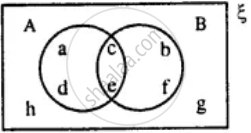Exercise 6 (E) | Q 1.2 | Page 75

From the given diagram find :
A' ∩ BExercise 6 (E) | Q 1.3 | Page 75

From the given diagram find :
A - BExercise 6 (E) | Q 1.4 | Page 75

From the given diagram find :
B - AExercise 6 (E) | Q 1.5 | Page 75

From the given diagram find :
(A ∪ B)'Exercise 6 (E) | Q 2 | Page 75

From the given diagram, find:
(i) A’
(ii) B’
(iii) A' ∪ B'
(iv) (A ∩ B)'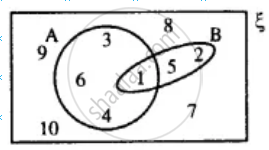Is A' ∪ B' = (A ∩ B)' ?

Also, verify if A' ∪ B' = (A ∩ B)'.

Exercise 6 (E) | Q 3 | Page 76

Use the given diagram to find:

(i) A ∪ (B ∩ C)

(ii) B - (A - C)

(iii) A - B

(iv) A ∩ B'

Is A ∩ B' = A - B?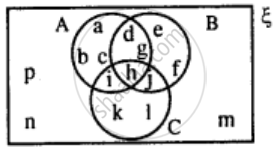Exercise 6 (E) | Q 4.1 | Page 76

Use the given Venn-diagram to find:
B - A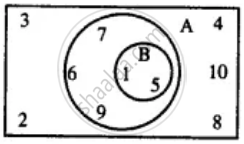Exercise 6 (E) | Q 4.2 | Page 76

Use the given Venn-diagram to find :
AExercise 6 (E) | Q 4.3 | Page 76

Use the given Venn-diagram to find :
B'Exercise 6 (E) | Q 4.4 | Page 76

Use the given Venn-diagram to find :
A ∩ BExercise 6 (E) | Q 4.5 | Page 76

Use the given Venn-diagram to find :
A ∪ BExercise 6 (E) | Q 5.1 | Page 76

Draw a Venn-diagram to show the relationship between two overlapping sets A and B. Now shade the region representing :
A ∩ B

Exercise 6 (E) | Q 5.2 | Page 76

Draw a Venn-diagram to show the relationship between two overlapping sets A and B. Now shade the region representing :
A ∪ B

Exercise 6 (E) | Q 5.3 | Page 76

Draw a Venn-diagram to show the relationship between two overlapping sets A and B. Now shade the region representing :
B - A

Exercise 6 (E) | Q 6.1 | Page 76

Draw a Venn-diagram to show the relationship between two sets A and B; such that A ⊆ B, Now shade the region representing :
A ∪ B

Exercise 6 (E) | Q 6.2 | Page 76

Draw a Venn-diagram to show the relationship between two sets A and B; such that A ⊆ B, Now shade the region representing :
B' ∩ A

Exercise 6 (E) | Q 6.3 | Page 76

Draw a Venn-diagram to show the relationship between two sets A and B; such that A ⊆ B, Now shade the region representing :
A ∩ B

Exercise 6 (E) | Q 6.4 | Page 76

Draw a Venn-diagram to show the relationship between two sets A and B; such that A ⊆ B, Now shade the region representing :
(A ∪ B)'

Exercise 6 (E) | Q 7.1 | Page 76

Two sets A and B are such that A ∩ B = Φ. Draw a venn-diagram to show the relationship between A and B. Shade the region representing :
A ∪ B

Exercise 6 (E) | Q 7.2 | Page 76

Two sets A and B are such that A ∩ B = Φ. Draw a venn-diagram to show the relationship between A and B. Shade the region representing :
(A ∪ B)'

Exercise 6 (E) | Q 7.3 | Page 76

Two sets A and B are such that A ∩ B = Φ. Draw a venn-diagram to show the relationship between A and B. Shade the region representing :
B - A

Exercise 6 (E) | Q 7.4 | Page 76

Two sets A and B are such that A ∩ B = Φ. Draw a venn-diagram to show the relationship between A and B. Shade the region representing :
B ∩ A'

Exercise 6 (E) | Q 8.1 | Page 76

State the sets representing by the shaded portion of following venn-diagram :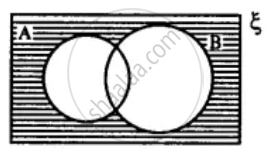Exercise 6 (E) | Q 8.2 | Page 76

State the sets representing by the shaded portion of following venn-diagram :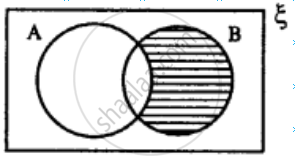Exercise 6 (E) | Q 8.3 | Page 76

State the sets representing by the shaded portion of following venn-diagram :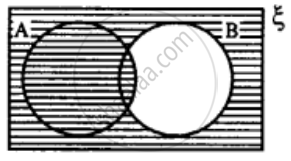Exercise 6 (E) | Q 9.1 | Page 76

In the given diagram, shade the region which represents the set given underneath the diagrams: (B - A)'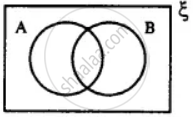Exercise 6 (E) | Q 9.2 | Page 76

In the given diagram, shade the region which represents the set given underneath the diagrams: (A ∩ B)'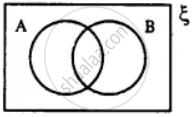Exercise 6 (E) | Q 9.3 | Page 76

In the given diagram, shade the region which represents the set given underneath the diagrams: (P ∩ Q)'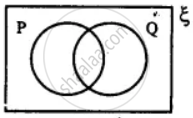Exercise 6 (E) | Q 10 | Page 76

From the given diagram, find :
(i) (A ∪ B) - C

(ii) B - (A ∩ C)

(iii) (B ∩ C) ∪ A

Verify :
A - (B ∩ C) = (A - B) ∪ (A - C)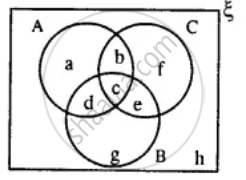Exercise 6 (E) | Q 11.1 | Page 76

Using the given diagram, express the following sets in the terms of A and B. {a, d}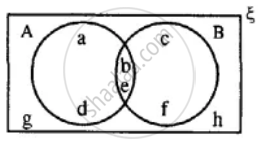Exercise 6 (E) | Q 11.2 | Page 76

Using the given diagram, express the following sets in the terms of A and B. {a, d, c, f}Exercise 6 (E) | Q 11.3 | Page 76

Using the given diagram, express the following sets in the terms of A and B. {a, d, c, f, g, h}Exercise 6 (E) | Q 11.4 | Page 76

Using the given diagram, express the following sets in the terms of A and B. {a, d, g, h}Exercise 6 (E) | Q 11.5 | Page 76

Using the given diagram, express the following sets in the terms of A and B. {g, h}## Solutions for Chapter 6: Sets

Exercise 6 (A)Exercise 6 (B)Exercise 6 (C)Exercise 6 (D)Exercise 6 (E)## Selina solutions for Concise Mathematics Class 8 ICSE chapter 6 - Sets

Shaalaa.com has the CISCE Mathematics Concise Mathematics Class 8 ICSE CISCE solutions in a manner that help students grasp basic concepts better and faster. The detailed, step-by-step solutions will help you understand the concepts better and clarify any confusion. Selina solutions for Mathematics Concise Mathematics Class 8 ICSE CISCE 6 (Sets) include all questions with answers and detailed explanations. This will clear students' doubts about questions and improve their application skills while preparing for board exams.

Further, we at Shaalaa.com provide such solutions so students can prepare for written exams. Selina textbook solutions can be a core help for self-study and provide excellent self-help guidance for students.

Concepts covered in Concise Mathematics Class 8 ICSE chapter 6 Sets are Concept of Sets, Representation of a Set, Cardinal Number of a Set, Types of Sets, Subset, Proper Subset, Number of Subsets and Proper Subsets of a Given Set, Super Set, Universal Set, Set Operations, Difference of Two Sets, Distributive Laws, Complement of a Set, Venn Diagrams.

Using Selina Concise Mathematics Class 8 ICSE solutions Sets exercise by students is an easy way to prepare for the exams, as they involve solutions arranged chapter-wise and also page-wise. The questions involved in Selina Solutions are essential questions that can be asked in the final exam. Maximum CISCE Concise Mathematics Class 8 ICSE students prefer Selina Textbook Solutions to score more in exams.

Get the free view of Chapter 6, Sets Concise Mathematics Class 8 ICSE additional questions for Mathematics Concise Mathematics Class 8 ICSE CISCE, and you can use Shaalaa.com to keep it handy for your exam preparation.